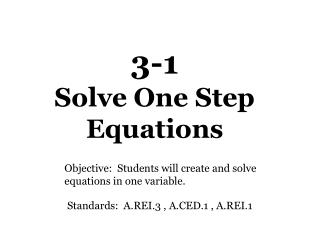# 3-1 Solve One Step Equations - PowerPoint PPT PresentationDownload Presentation3-1 Solve One Step Equations

3-1 Solve One Step EquationsDownload Presentation## 3-1 Solve One Step Equations

- - - - - - - - - - - - - - - - - - - - - - - - - - - E N D - - - - - - - - - - - - - - - - - - - - - - - - - - -
##### Presentation Transcript

1. 3-1 Solve One Step Equations Objective: Students will create and solve equations in one variable. Standards: A.REI.3 , A.CED.1 , A.REI.1

2. Inverse Operations: Two operations that undo each other. Examples: Addition & Subtraction Multiplication & Division Equivalent Equations: Equations that have the same solution(s). Vocabulary

3. Solve: x + 9 = 3 Example 1 Isolate the variable. Get x by itself by doing the opposite operation. -9 -9 What you do to ONE SIDE, you must do to the OTHER SIDE of the equation to keep it balanced. x = -6

4. To justify steps must use PROPERTIES Solve. Justify your steps: x - 2 = 11 Example 2 Use 2-column proof to do this x – 2 = 11 Given +2 +2 Addition property of Equality (APOE) x = 13 Simplify

5. Example 3 To justify steps must use PROPERTIES Solve. Justify your steps: -12 = x – 4 Use 2-column proof to do this -12 = x – 4 Given x – 4 = -12 Symmetric (can SWITCH over = sign) +4 +4 APOE x = -8 Simplify

6. Solve: -4x = -28 Example 4 Isolate the variable. Opposite of Multiplication is DIVISION. -4 -4 x = 7

7. Example 5 To justify steps must use PROPERTIES Solve. Justify your steps: -40 = 5x Use 2-column proof to do this -40 = 5x Given 5x = -40 Symmetric (can SWITCH over = sign) 5 5 Mult. Prop. of Equality (MPOE) x = -8 Simplify

8. Solve: x = 7 3 WELCOME Example 6 Isolate the variable. Opposite of Division is MULTIPLICATION. (3) (3) Must do the same operation to BOTH sides of the equation to keep it balanced. x = 21

9. Example 7 Solve. Justify your steps: Isolate the variable. Multiply by the RECIPROCAL of the fraction. 5 3 5 3 Given Mult. Prop. of Equality (MPOE) Simplify 1 x = -15

10. Write and solve an equation for the following situation: You are working on a banner for Friday’s pep rally. The length of the banner is 3 times the width. The length is 15 feet. What is the width? Example 8 What do you know? W = width L = 3 times width 15 = length 3w = 15 3 3 w = 5 feet

11. MUST SHOW ALL STEPS!!NO STEPS = NO CREDIT!!

12. Homework Section 3-1 Page 137 – 138 Solve & PROVE: 6 – 11, 20 – 25, 36 – 39, 46 – 47 ; Follow Directions: 30, 53 - 56

13. 3-2Solve Two Step Equations Objective: Students will create and solve equations in one variable. Standards: A.REI.3 , A.CED.1 , A.REI.1

14. Solve: Example 1 Isolate the variable. Opposite of Subtraction is ADDITION. +3 +3 x = 5 4 (4) (4) Opposite of Division is MULTIPLICATION. x = 20

15. Example 2 To justify steps must use PROPERTIES Solve. Justify your steps: 20 = 8y – 12 Use 2-column proof to do this 20 = 8y - 12 Given 8y -12 = 20 Symmetric (can SWITCH over = sign) +12 +12 Add. Prop. of Equality (APOE) 8y = 32 Simplify 8 8 Mult. Prop. of Equality (MPOE) y = 4 Simplify

16. Solve and Prove: 5b – 7b = 4 Example 3 Combine Like Terms FIRST! SAME SIDE COMBINE!!! Given 5b – 7b = 4 -2b = 4 Combine like terms -2 -2 Isolate the Variable. Opposite of Multiplication is DIVISION. Mult. Prop. Of Equality (MPOE) b = -2 Simplify

17. To rent a booth at the county fairgrounds costs \$42 per day plus a one time equipment fee of \$85. Find the number of days Mr. Smith rented a booth if he paid a total of \$337. Example 4 “per” means MULTIPLY. This is where your “x” goes! STEP 1: Write the equation. 42x 337 + 85 = STEP 2: Solve the equation. -85 -85 42x = 252 42 42 x = 6 days

18. Homework Section 3-2 Page 144 – 145 SOLVE & PROVE: 6 – 20, 28 - 30 Follow Directions: 22, 37, 38